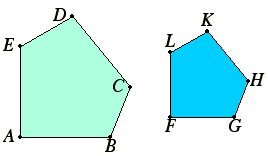# Definition 1

Similar rectilinear figures are such as have their angles severally equal and the sides about the equal angles proportional.

## Guide

The words in this definition do not quite express its entire intent. It is apparent from its use that the notion of similarity assumes a specific correspondence of consecutive vertices and sides. Consider, for instance, pentagons.In order for the pentagons ABCDE and FGHKL to be similar, it is required that
1. corresponding angles taken in order are equal, that is, A = F, B = G, C = H, D = K, and E = L, and
2. the sides about their equal angles are proportional in the same order:

 EA:AB = LF : FG, AB:BC = FG : GH, BC:CD = GH : HK, CD:EF = HK : KL, and EA:AB = KL : LF.

It wouldn’t be allowed, for instance, if the angles of one figure were equal to the angles of the other, but in some haphazard order. And it wouldn’t be allowed for the orders of the terms in the proportions to be permuted, or inverted, for instance, the second proportion could not be AB:BC = GH : FG.

#### Use of this definition

Propositions VI.4 and VI.5 give two criteria for two triangles to be similar. Proposition VI.4 says that condition 1 implies similarity, while VI.5 says condition 2 implies similarity. Proposition VI.6 is a side-angle-side similarity theorem, and VI.7 is a side-side-angle similarity theorem. Many of the other propositions in this and later books involve similarity in one way or another.